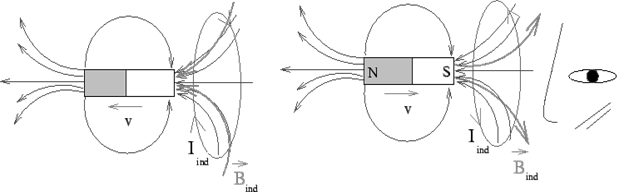Electromagnetic Induction: We have seen that studies made by Oersted, Biot-Savart and Ampere showed that an electric current produces a magnetic field. Michael Faraday wanted to explore if this phenomenon is reversible in the sense whether a magnetic field could be source for a current in a conductor. However, no current was found when a conductor was placed in a magnetic field. Faraday and (Joseph) Henry, however, found that if a current loop was placed in a time varying magnetic field or if there was a relative motion between a magnet and the loop a transient current was established in the conducting loop. They concluded that the source of the electromotive force driving the current in the conductor is not the magnetic field but the changing magnetic flux associated with the loop. The change in flux could be effected by (i) a time varying magnetic field or by (ii) motion of the conductor in a magnetic field or (iii) by a combined action of both of these. The discovery is a spectacular milestone in the sense that it led to important developments in Electrical engineering like invention of transformer, alternator and generator.
Shortly after Faraday's discovery, Heinrich Lenz found that the direction of the induced current is such that it opposes the very cause that produced the induced current (i.e. the magnetic field associated with the induced current opposes the change in the magnetic flux which caused the induced current in the first place). Lenz's law is illustrated in the followingIn the figures the loops are perpendicular to the plane of the page. The direction of induced current is as seen towards the loop from the right. Note that the magnetic field set up by the induced current tends to increase the flux in the case where the magnet is moving away from the loop and tends to decrease it in the case where it is moving towards the loop,
Mathematically, Faraday's law is stated thus : the electromotive force is proportional to the rate of change of magnetic flux. In SI units, the constant of proportionality is unity.whereis the flux associated with the circuit and the minus sign is a reminder of the direction of the current as given by Lenz's law. If the loop containsturns, the equation becomesThough the flux is a scalar, one can fix its sign by considering the sign of the area vector which is fixed by the usual right hand rule. The dot product ofandthen has a sign.